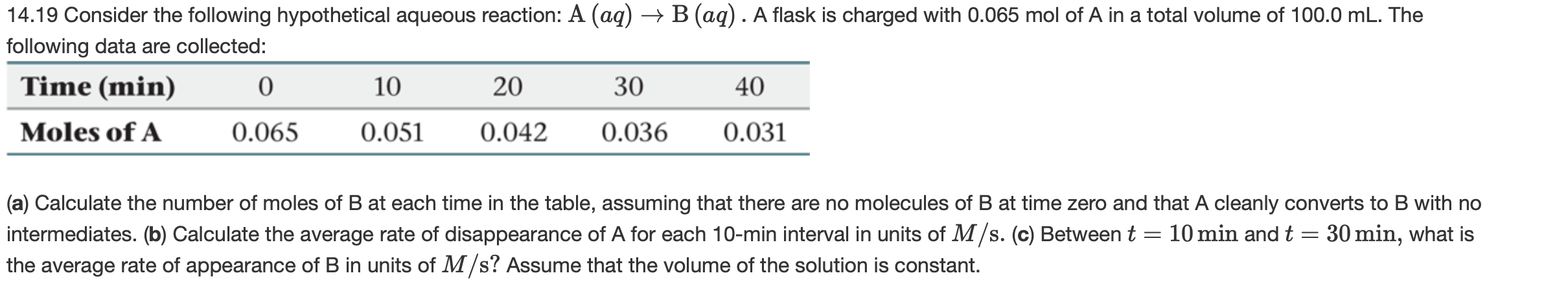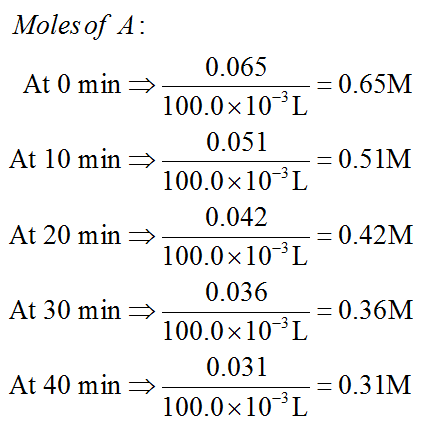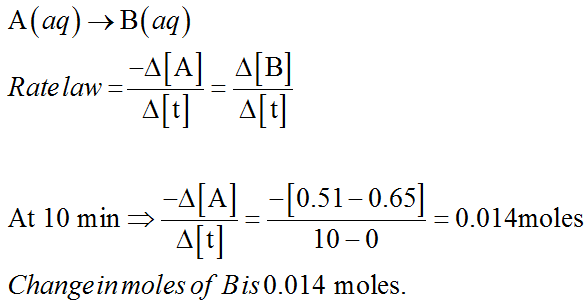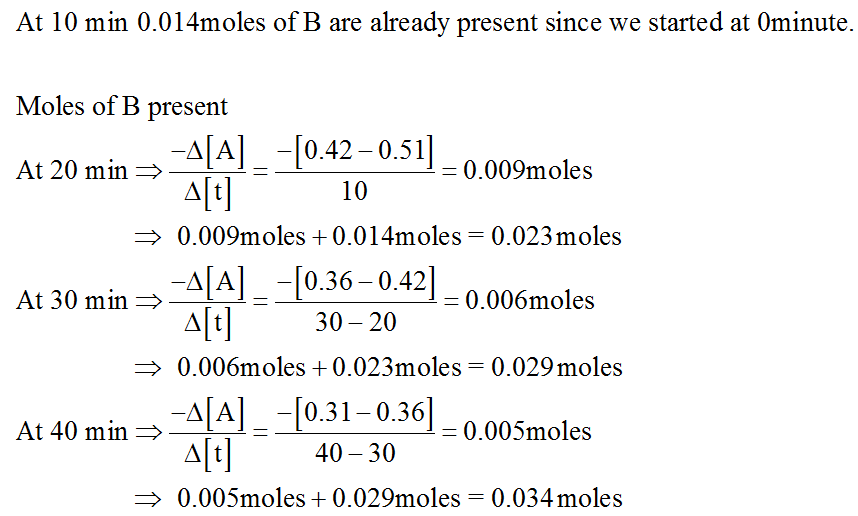# → B (aq). A flask is charged with 0.065 mol of A in a total volume of 100.0 mL. The14.19 Consider the following hypothetical aqueous reaction: A (aq)following data are collected:Time (min)102030400.031Moles of A0.0650.0510.0420.036(a) Calculate the number of moles of B at each time in the table, assuming that there are no molecules of B at time zero and that A cleanly converts to B with nointermediates. (b) Calculate the average rate of disappearance of A for each 10-min interval in units of M/s. (c) Between tthe average rate of appearance of B in units of M/s? Assume that the volume of the solution is constant.10 min and t = 30 min, what is

Question
48 viewshelp_outlineImage Transcriptionclose→ B (aq). A flask is charged with 0.065 mol of A in a total volume of 100.0 mL. The 14.19 Consider the following hypothetical aqueous reaction: A (aq) following data are collected: Time (min) 10 20 30 40 0.031 Moles of A 0.065 0.051 0.042 0.036 (a) Calculate the number of moles of B at each time in the table, assuming that there are no molecules of B at time zero and that A cleanly converts to B with no intermediates. (b) Calculate the average rate of disappearance of A for each 10-min interval in units of M/s. (c) Between t the average rate of appearance of B in units of M/s? Assume that the volume of the solution is constant. 10 min and t = 30 min, what is fullscreen
check_circle

Step 1

a)

At time 0 minute the number of moles of B is 0.

Molarity of A = moles of A/volume of solution in L.Step 2

Number of moles of B at given time intervals are determined as follows,Step 3...

### Want to see the full answer?

See Solution

#### Want to see this answer and more?

Solutions are written by subject experts who are available 24/7. Questions are typically answered within 1 hour.*

See Solution
*Response times may vary by subject and question.
Tagged in

### Chemistry